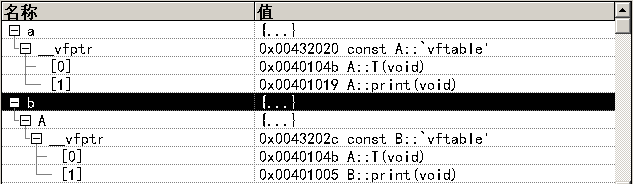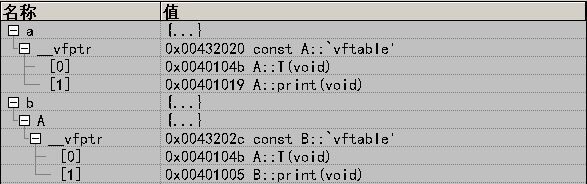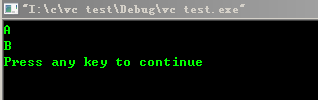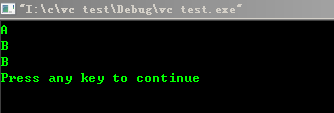# C++中为什么要用虚函数、指针或引用才能实现多态？

class A
{
public:
virtual void print()
{cout<<"A"<<endl;}
};

class B:public A
{
public:
void print()
{cout<<"B"<<endl;}
};
int main()
{
A a;
B b;

A *pa = &b;//能实现多态
pa->print();

a = b; //不能实现多态,为什么?
a.print();

return 0;
}

	class A
{
public:
virtual void T(){}
virtual void print()
{cout<<"A"<<endl;}
};
class B:public A
{
public:
void print()
{cout<<"B"<<endl;}
};
int main()
{
A a;
B b;
a = b;
a.print();                         //通过对象直接访问
b.print();
return 0;
}A& operator = (const B& b)
{
*(int *)this=*(int *)&b;
return *this;
}A *pa = &a;
pa->print();1.默认的赋值运算符并不会操作虚函数表。

2.要实现多态，必须使用指针或者引用。